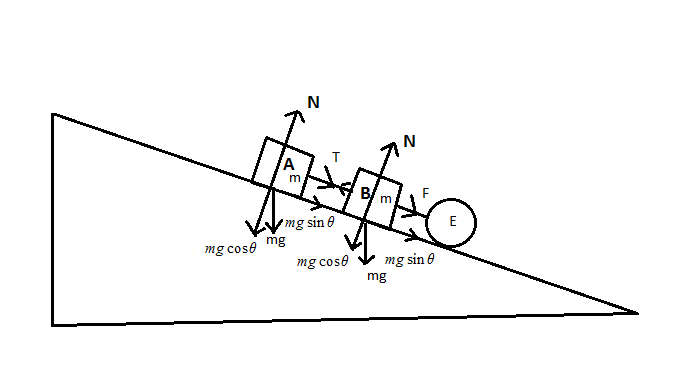# An engine is used to pull a train of two cars out of a mine. The floor of the mine slopes upward at an angle of 30 degrees. Each car has a mass of 10^4 kg and travels without friction. The engine can exert a max force of 1.5 * 10^5 N on car Aa) The engine first accelerates the cars from rest by exerting its maximum force. What is the acceleration of the cars?b) Once the train has reached a reasonable speed, the engineer throttles back so they continue at a constant speed. Now what force does the engine exert on car A?c) What is the tension in the massless, straight chain connecting cars A and B while they are traveling at a constant speed?

Question
99 views

An engine is used to pull a train of two cars out of a mine. The floor of the mine slopes upward at an angle of 30 degrees. Each car has a mass of 10^4 kg and travels without friction. The engine can exert a max force of 1.5 * 10^5 N on car A
a) The engine first accelerates the cars from rest by exerting its maximum force. What is the acceleration of the cars?
b) Once the train has reached a reasonable speed, the engineer throttles back so they continue at a constant speed. Now what force does the engine exert on car A?
c) What is the tension in the massless, straight chain connecting cars A and B while they are traveling at a constant speed?

check_circle

Step 1

It is given that the engine is pulling two cars of equal mass out of a mine. The floor of mine is a frictionless upward slope.

Let car A connected to the engine has acceleration aA and car B has acceleration aB

We would draw a free body diagram.Step 2

The force exerted by engine on car A + The component of weight of Car A along the slope would be responsible for the acceleration of car A.

Write this in form equation and substitute the values.

Step 3

When car A would accelerate, it would pull car B. We...

### Want to see the full answer?

See Solution

#### Want to see this answer and more?

Solutions are written by subject experts who are available 24/7. Questions are typically answered within 1 hour.*

See Solution
*Response times may vary by subject and question.
Tagged in
SciencePhysics

### Kinematics